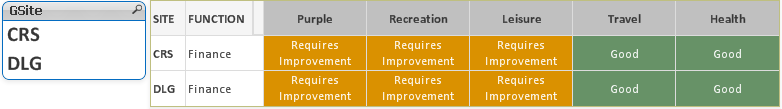# New to QlikView

Discussion board where members can get started with QlikView.

Highlighted
Contributor II

## Pivot and Aggr functions

Hi all,

Good afternoon! I have a two-dimensional pivot table with AGGR, IF AND COUNT expressions.

The two dimensions are Gsite and GFunction. The Finance 'Function' is located at two Sites (CRS, DLG) and I wanted to see the performance of 'Finance' at both the sites. I am using the following expression.

=aggr(nodistinct if(count({<GRating = {'10'}>} GQuestions)>=3 and count({<GRating = {'<=6'}>}GQuestions)=0, 'Outstanding',
if(count({<GRating = {'>=7 <=9'}>}GQuestions)=count(GQuestions), 'Good',
if(count({<GRating = {'<=3'}>}GQuestions)>=3, 'Inadequate',
if(count({<GRating = {'<=6'}>}GQuestions)>=3, 'Requires Improvement',
if(count({<GRating = {'<=3'}>}GQuestions)>=2, 'Requires Improvement', 'Good'))))), GFunction, GDomain)

When I DO NOT apply any filters, I am getting the same result for both the sites. Please see screen shot below. This is wrong because CRS and DLG sites have different values.When I apply a filter CRS or DLG, the value changes. see screen shot below.Could someone please tell me what is going wrong and how can I show the correct values for both the Sites without applying the filter?

I have attached the QVW file and the spreadsheet.

Thanks!

Karthik

Tags (2)
1 Solution

Accepted SolutionsMVP

## Re: Pivot and Aggr functions

Hi,

Include the GSite in Aggregation.

=aggr(nodistinct if(count({<GRating = {'10'}>} GQuestions)>=3 and count({<GRating = {'<=6'}>}GQuestions)=0, 'Outstanding',

if(count({<GRating = {'>=7 <=9'}>}GQuestions)=count(GQuestions), 'Good',

if(count({<GRating = {'<=3'}>}GQuestions)>=3, 'Inadequate',

if(count({<GRating = {'<=6'}>}GQuestions)>=3, 'Requires Improvement',

if(count({<GRating = {'<=3'}>}GQuestions)>=2, 'Requires Improvement',  'Good'))))), GFunction, GDomain,GSite)

Regards,

Kaushik Solanki

2 RepliesMVP

## Re: Pivot and Aggr functions

Hi,

Include the GSite in Aggregation.

=aggr(nodistinct if(count({<GRating = {'10'}>} GQuestions)>=3 and count({<GRating = {'<=6'}>}GQuestions)=0, 'Outstanding',

if(count({<GRating = {'>=7 <=9'}>}GQuestions)=count(GQuestions), 'Good',

if(count({<GRating = {'<=3'}>}GQuestions)>=3, 'Inadequate',

if(count({<GRating = {'<=6'}>}GQuestions)>=3, 'Requires Improvement',

if(count({<GRating = {'<=3'}>}GQuestions)>=2, 'Requires Improvement',  'Good'))))), GFunction, GDomain,GSite)

Regards,

Kaushik Solanki

Contributor II

## Re: Pivot and Aggr functions

Great, thanks Kaushik!